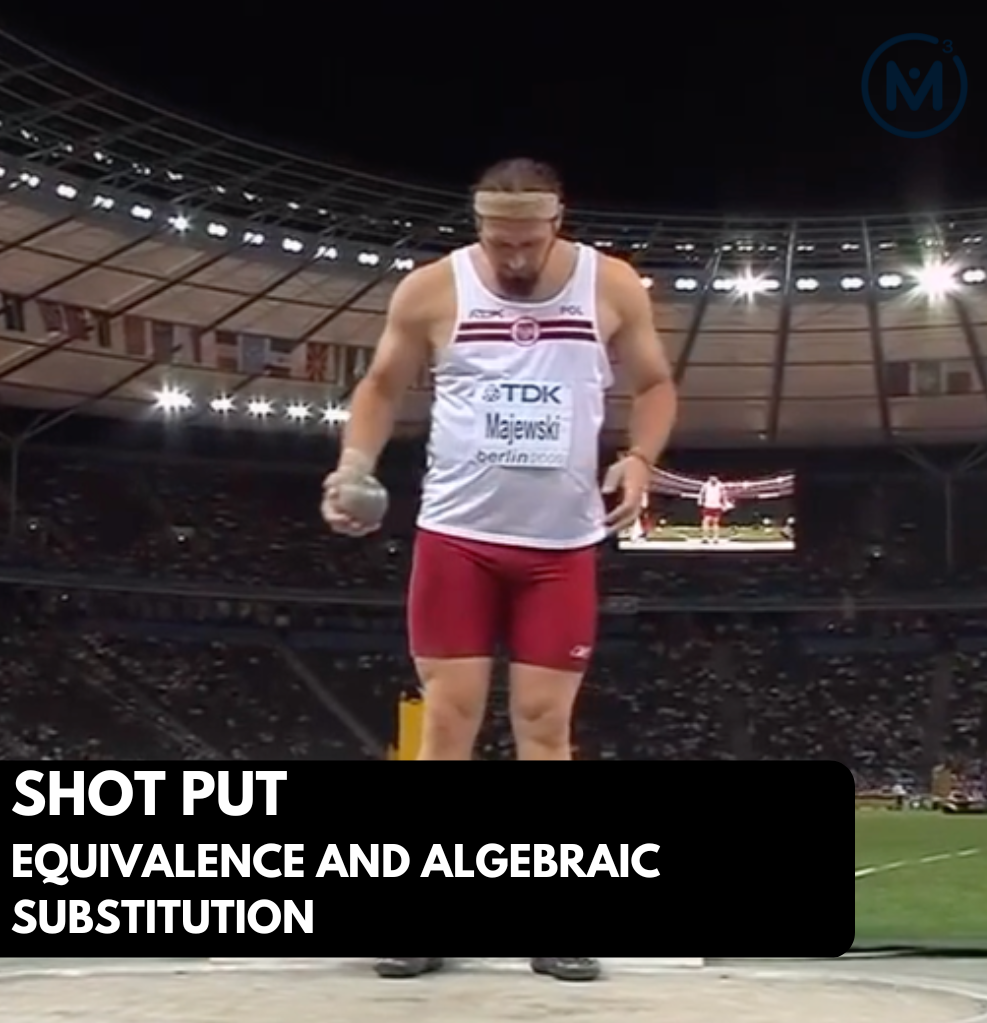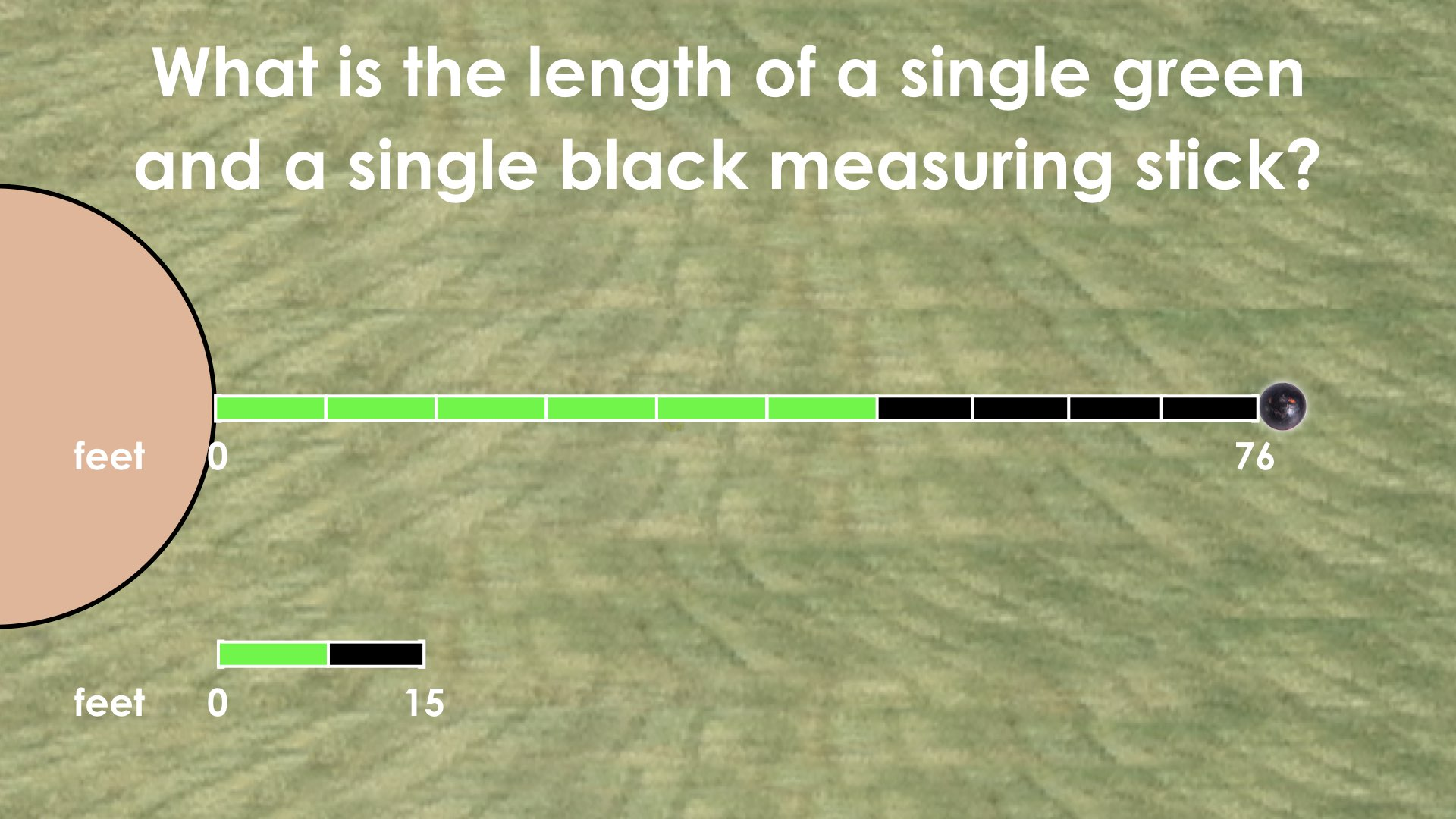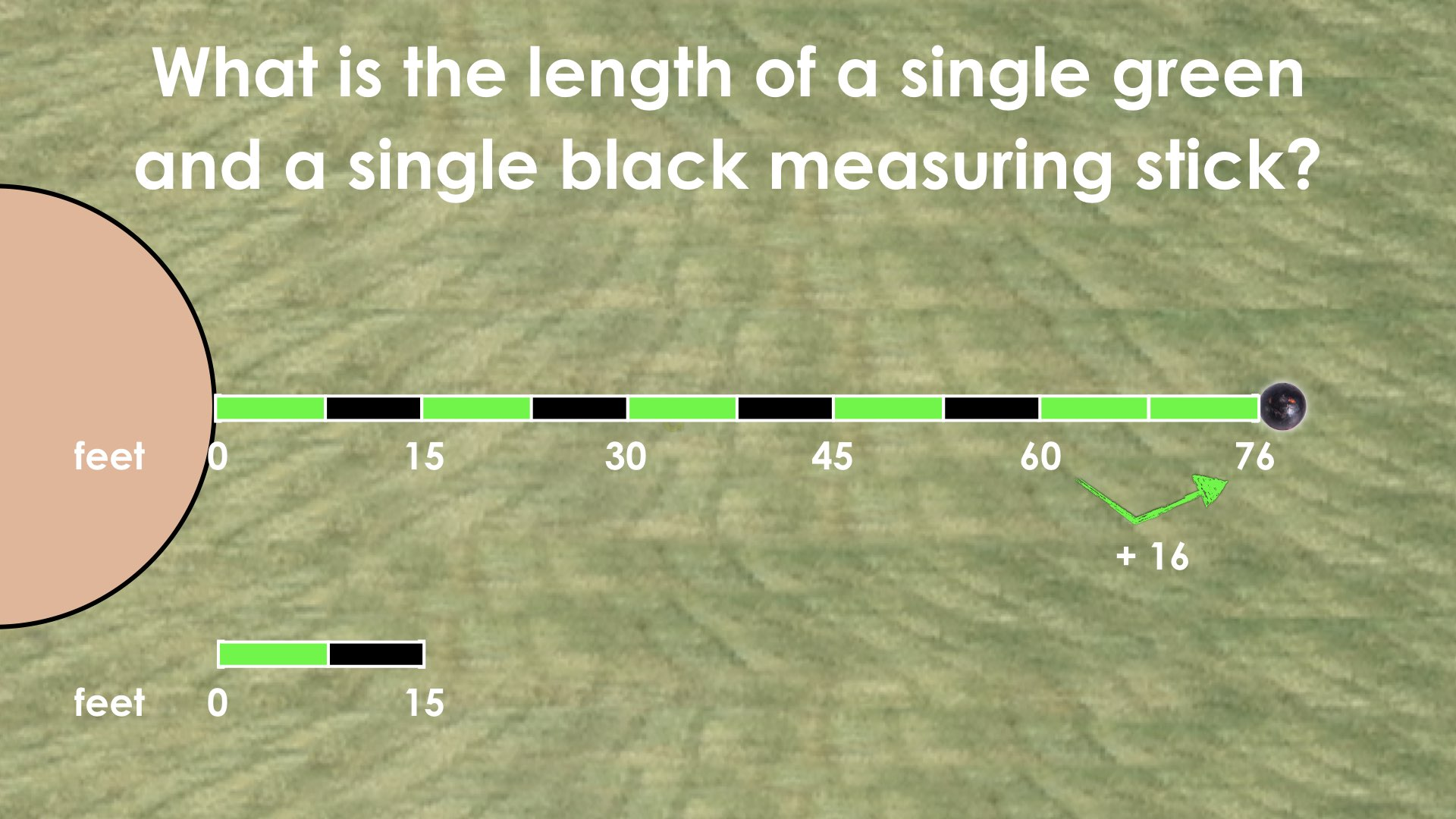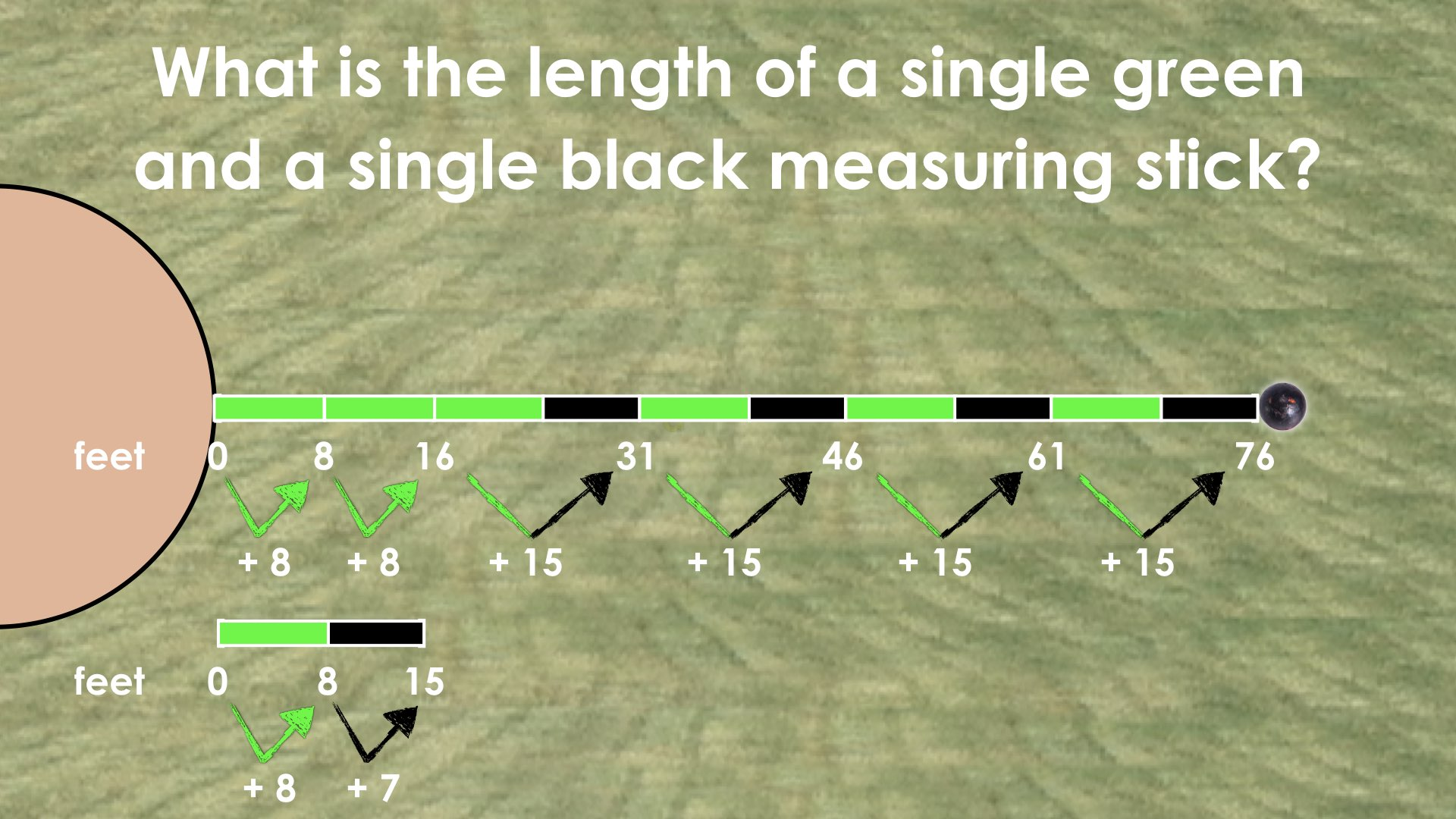## SHOT PUT [DAY 3]

### EQUIVALENCE AND ALGEBRAIC SUBSTITUTION

Student will explore solving equations using the idea of equivalence and substitution.

## Intentionality & Unit Overview### Access each lesson from this unit using the navigation links below

Students will explore solving equations using the idea of equivalence and substitution.

## Intentionality…

Today, students will have an opportunity to reason through an investigation extending the shot put context. The tasks involve measuring different shot put throws with two different length measuring sticks. In this scenario, where the total length of each throw and number of measuring sticks is known, but the length of each measuring stick is unknown allows students to solve a two-variable system of linear equations using mathematical models such as the number line and/or double number line.

This scenario builds off of our day 2 understanding of partitive division meaning the number of sticks (i.e.: parts) are known and the length of each stick (i.e.: rate or quota) are unknown. Although we are not necessarily using partitive division, the big ideas are still prevalent.

They will also explore big ideas including the following:

• There are two types of division; partitive and quotative.
• Each side of an equation can be thought of as a single unit, where both sides are equivalent.
• Quantities can be decomposed and reassociated to create new units that can be substituted for equivalent quantities.
• The algebraic strategy of substitution can assist in solving for unknown quantities in an equation.

## Walk Through Video

For today’s Math Talk, we will continue to work with the Shot Put context from earlier in this unit.

In this string of related problems, we will be providing students with a particular number of feet for shot put throws and the number of sticks used to measure the throw length exactly without having to add or subtract additional feet. The goal is to determine the length of each measuring stick. Since we are dividing a quantity of feet (i.e.: shot put throw distance) into parts (i.e.: a given number of measuring sticks) to reveal the length of each measuring stick (i.e.: the rate), these are partitive division scenarios.

How long would each measuring stick be if…

… a 12 foot throw was measured exactly with 4 measuring sticks?

… a 24 foot throw was measured exactly with 4 measuring sticks?

… a 24 foot throw was measured exactly with 8 measuring sticks?

… a 48 foot throw was measured exactly with 16 measuring sticks?

A second possible string for another day or if needed:

How long would each measuring stick be if…

… a 16 foot throw was measured exactly with 4 measuring sticks?

… a 48 foot throw was measured exactly with 12 measuring sticks?

… a 24 foot throw was measured exactly with 6 measuring sticks?

Consider modelling student thinking on a double number line.

As you work through this math talk, consider the implications on the quotient (i.e.: the length of each measuring stick) if we double the length of the throw, double the number of sticks used for measuring, or double both.

Are students able to notice any patterns?

Big ideas:

Equivalence; that 12 feet divided partitively by 4 measuring sticks is equivalent to 24 feet divided by 8 measuring sticks.

Facilitator Notes:

Note that on day 2, our math talk focused on scaling both sides of the equation down, today’s math talk is focusing on scaling up and down our initial partitive division expression.

The purpose is to reveal other partitive division expressions and determine when the rate (length per stick) will be equivalent, when it will not, and to expose the impact that scaling the dividend and/or the divisor has on the quotient in order to make generalizations.

## Walk Through Video

If you’d like to be walked through this math talk to gain a better understanding of the intentionality behind it, watch the following video:

## Craft A Productive Struggle: Prompt

Pose the following question:
A shot put was thrown and landed at a distance of 76 feet. The officials measured the throw using 6 green measuring sticks and 4 black measuring sticks. The total length of 1 green and 1 black measuring combined is 15 feet. Determine the length of a single green and a single black measuring stick.
We recommend that you try to avoid sharing a visual with students upfront to see what students can do on their own. This is a great opportunity to see if students are able to visualize this situation in their minds and successfully model the scenario without being given a visual to start with. Try posing purposeful questions that will help students draw or build the situation. This might look like jumps on a number line, labelling 6 of the jumps as “g” for the length of a green stick and “b” on 4 of the jumps representing the length of a black stick.## Facilitator Notes

As students are working, encourage them to model the scenario representing 6 green and 4 black measuring sticks for a total length of 76 feet. A number line may be a helpful suggestion, however be sure not to funnel student thinking too forcefully. Consider asking students purposeful questions that will encourage students to consider the relationship between 1 green and 1 black measuring stick and how this information might be helpful for solving? As the facilitator, you should be aware that every unit of 1 green and 1 black measuring stick represents a total of 15 feet. With this in mind, students can make jumps of 15 using 1 green and 1 black measuring sticks until they run out of black measuring sticks. While it is important for the facilitator to know this, please do not suggest students use this strategy. Rather, think of questions that might allow students to discover this idea for themselves.

## While Students Are Productively Struggling…

Login/Join to access the entire Teacher Guide, downloadable slide decks and printable handouts for this lesson and all problem based units.

## Student Approach #1: Number Line Using Equivalence and Substitution

Login/Join to access the entire Teacher Guide, downloadable slide decks and printable handouts for this lesson and all problem based units.

## Student Approach #2: Pictorial Representation Using Associative Property and Substitution

Login/Join to access the entire Teacher Guide, downloadable slide decks and printable handouts for this lesson and all problem based units.

## Student Approach #3: Symbolic Representation Using Associative and Substitution

Login/Join to access the entire Teacher Guide, downloadable slide decks and printable handouts for this lesson and all problem based units.

## Reveal

After consolidating learning using student generated solution strategies and by extending their thinking intentionally, we can share what really happened by showing the following video/image revealing the scenario unfolding.

If your students are at a place where they are already modelling symbolically or they are ready to be nudged there, this silent solution video showing how the visual double number line model can be connected to the symbolic representation using equations might be helpful:Length of a green measuring stick, g = 8 feet

Length of a black measuring stick, b = 7 feet

## Consolidation

Login/Join to access the entire Teacher Guide, downloadable slide decks and printable handouts for this lesson and all problem based units.

### Facilitator Notes

Login/Join to access the entire Teacher Guide, downloadable slide decks and printable handouts for this lesson and all problem based units.

## Reflect and Consolidation Prompts

Provide students an opportunity to reflect on their learning by offering this consolidation prompt to be completed independently.

### Consolidation Prompt #1:

Login/Join to access the entire Teacher Guide, downloadable slide decks and printable handouts for this lesson and all problem based units.

### Consolidation Prompt #2:

Login/Join to access the entire Teacher Guide, downloadable slide decks and printable handouts for this lesson and all problem based units.

Login/Join to access the entire Teacher Guide, downloadable slide decks and printable handouts for this lesson and all problem based units.

## Educator Discussion Area

Login/Join to access the entire Teacher Guide, downloadable slide decks and printable handouts for this lesson and all problem based units.

## Explore Our 60+ Problem Based Units

This Make Math Moments Lesson was designed to spark curiosity for a multi-day unit of study with built in purposeful practice, number talks and extensions to elicit and emerge strategies and mathematical models.

Dig into our other units of study and view by concept continuum, grade or topic!